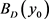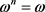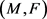# 从Finsler流形到Riemann流形的调和映射梯度估计Gradient Estimate for Harmonic Maps from Finsler Manifolds to Riemannian Manifolds

DOI: 10.12677/PM.2020.102013, PDF, HTML, XML, 下载: 296  浏览: 514

Abstract: In this paper, we study gradient estimate for harmonic maps from Finsler manifolds to Riemannian manifolds. As an application, we obtain Liouville type theorem of harmonic maps from a weak Landsberg manifold to a Cartan-Hadamard manifold. Moreover, we generalize the Liouville type theorem to a regular ball of Riemannian manifold.

1. 引言

1975年，丘成桐  建立了非负Ricci曲率完备Riemann流形上正调和函数的梯度估计，并给出了相应的Liouville型定理。之后，郑绍远  和H.I.Choi  分别推导了从Ricci曲率有下界的完备Riemann流形到Cartan-Hadamard流形和正则球的调和映射的梯度估计，同时得到了相应的Liouville型定理。他们主要运用了Bochner技巧和最大值原理。

$E\left(\phi \right)=\frac{1}{c}{\int }_{SM}\frac{1}{2}{|d\phi |}^{2}\Pi ,$

$\tau \left(\phi \right)=trace\nabla d\phi +〈d\phi ,J〉\in \Gamma \left({\phi }^{*}TN\right),$ (1)

$\begin{array}{c}\frac{1}{2}{\Delta }^{H}{|d\phi |}^{2}={|{\nabla }^{H}d\phi |}^{2}+〈{\nabla }^{H}\tau \left(\phi \right),d\phi 〉+〈d\phi \left(\stackrel{˜}{R}\right),d\phi 〉\\ \text{\hspace{0.17em}}\text{\hspace{0.17em}}-\underset{i,j=1}{\overset{m}{\sum }}\stackrel{^}{R}\left(d\phi \left({e}_{i}\right),d\phi \left({e}_{j}\right),d\phi \left({e}_{i}\right),d\phi \left({e}_{j}\right)\right)-〈d\phi ,\nabla J\otimes d\phi 〉.\end{array}$

$\stackrel{˜}{R}\ge -{k}_{1},$

$\underset{x\in {\Omega }_{R}}{\mathrm{max}}{|d\phi |}^{2}\le \left(\frac{{C}_{1}}{R}+{k}_{1}\right){b}_{R}^{2}.$ (2)

$\stackrel{¯}{\underset{R\to +\infty }{\mathrm{lim}}}\frac{1}{\sqrt{R}}\mathrm{sup}\left\{\rho \circ \phi \left(x\right)|x\in {\Omega }_{2R}\right\}=0,$

${k}_{2}$${k}_{2}\ge 0$，若落在 ${y}_{0}\in N$ 的割迹之内且 $D<\frac{\pi }{2\sqrt{{k}_{2}}}$，则我们称 ${B}_{D}\left({y}_{0}\right)$ 是Rieman流形N的正则球，当 ${k}_{2}=0$ 时，我们要求 $D<+\infty$

$\stackrel{˜}{R}\ge -{k}_{1},$

$\underset{x\in {\Omega }_{R}}{\mathrm{max}}{|d\phi |}^{2}\le {C}_{2}\left({k}_{1}+\frac{1}{R}\right)\text{.}$ (3)

2. 预备知识

i) 正齐性： $F\left(x,\lambda y\right)=\lambda F\left(x,y\right),\forall \lambda >0$

ii) 光滑性： ${F|}_{TM\\left\{0\right\}}$${C}^{\infty }$ 的；

iii) 正定性：对于任意向量 $y\in TM\\left\{0\right\}$

${g}_{ij}\left(x,y\right)=\frac{1}{2}\frac{{\partial }^{2}{F}^{2}}{\partial {y}^{i}\partial {y}^{j}}\left(x,y\right)=\frac{1}{2}{\left({F}^{2}\right)}_{{y}^{i}{y}^{j}}\text{.}$

$g=\underset{i,j=1}{\overset{m}{\sum }}{g}_{ij}\left(x,y\right)d{x}^{i}\otimes d{x}^{j},$

$\omega =\underset{i=1}{\overset{m}{\sum }}\frac{\partial F}{\partial {y}^{i}}d{x}^{i}\in \Gamma \left({\pi }^{*}{T}^{*}M\right),$

$l=\underset{i=1}{\overset{m}{\sum }}\frac{{y}^{i}}{F}\frac{\partial }{\partial {x}^{i}}\in \Gamma \left({\pi }^{*}TM\right).$

Cartan张量C是定义在 ${\pi }^{\text{*}}TM$ 上的三阶对称张量：

$C=\underset{i,j,k=1}{\overset{m}{\sum }}{C}_{ijk}\left(x,y\right)d{x}^{i}\otimes d{x}^{j}\otimes d{x}^{k},$

$\eta =\underset{i,j,k=1}{\overset{m}{\sum }}{C}_{ijk}{g}^{jk}d{x}^{i},$

$\left(M,F\right)$ 是m维Finsler流形，由于l是单位长的向量场，因此总存在 ${\pi }^{\text{*}}TM$ 上的局部正交标架场 $\left\{{e}_{i}\right\}$ 使得 ${e}_{n}=l$，它的对偶标架场记为 $\left\{{\omega }^{i}\right\}$。在此标架下陈联络 $\nabla$ 的结构方程可表为：

$d{\omega }^{i}=\underset{j=1}{\overset{m}{\sum }}{\omega }^{j}\wedge {\omega }_{j}^{i},\text{\hspace{0.17em}}\text{\hspace{0.17em}}{\omega }_{j}^{i}+{\omega }_{i}^{j}=-2\underset{\lambda =1}{\overset{m-1}{\sum }}{H}_{ij\lambda }{\omega }_{n}^{\lambda }$ (4)

${\Omega }_{j}^{i}=\underset{k,s=1}{\overset{m}{\sum }}\frac{1}{2}{R}_{jks}^{i}{\omega }^{k}\wedge {\omega }^{s}+\underset{k=1}{\overset{m}{\sum }}\underset{\lambda =1}{\overset{m-1}{\sum }}{P}_{jk\lambda }^{i}{\omega }^{k}\wedge {\omega }_{n}^{\lambda }.$

$R={R}_{jks}^{i}{\omega }^{j}\otimes {\omega }^{k}\otimes {\omega }^{s}\otimes {e}_{i},$ (5)

$\stackrel{˜}{R}\left(X,Y\right)=\frac{1}{2}tr\left[〈R\left(X,\cdot \right)\cdot ,Y〉+〈R\left(Y,\cdot \right)\cdot ,X〉\right].$ (6)

Landsberg曲率L和平均Landsberg曲率J分别定义为：

$L=-{\nabla }_{l}C,\text{\hspace{0.17em}}\text{\hspace{0.17em}}J=-{\nabla }_{l}\eta .$

$G=\underset{i=1}{\overset{m}{\sum }}{\omega }^{i}\otimes {\omega }^{i}+\underset{\alpha =1}{\overset{m-1}{\sum }}{\omega }_{n}^{\alpha }\otimes {\omega }_{n}^{\alpha }.$

$TSM=HSM\oplus VSM,$

$\left(N,h\right)$ 是Riemann流形， $\left\{{\theta }^{a}\right\}$${T}^{\text{*}}N$ 上的局部正交标架场，它的对偶向量场记为 $\left\{{v}_{a}\right\}$。Levi-Civita

$d{\theta }^{a}={\theta }^{b}\wedge {\theta }_{b}^{a},\text{\hspace{0.17em}}\text{\hspace{0.17em}}{\theta }_{b}^{a}+{\theta }_{a}^{b}=0,\text{\hspace{0.17em}}\text{\hspace{0.17em}}d{\theta }_{b}^{a}={\theta }_{b}^{c}\wedge {\theta }_{c}^{a}+\frac{1}{2}{\stackrel{^}{R}}_{bcd}^{a}{\theta }^{c}\wedge {\theta }^{d}$ (7)

$\phi :M\to N$ 是光滑映射。它在SM上的提升仍然记为 $\phi$，能量定义为：

$E\left(\phi \right)=\frac{1}{c}{\int }_{SM}\frac{1}{2}{|d\phi |}^{2}\Pi ,$

$f:SM\to R$ 是光滑函数。水平Laplace算子定义为

${\Delta }^{H}f=di{v}_{G}\left({d}^{H}f\right)={g}^{ij}\left(\frac{{\delta }^{2}f}{\delta {x}^{i}\delta {x}^{j}}-\frac{\delta f}{\delta {x}^{k}}{\Gamma }_{ij}^{k}+\frac{\delta f}{\delta {x}^{k}}{L}_{ij}^{k}\right).$ (8)

$\frac{\delta }{\delta {x}^{i}}=\frac{\partial }{\partial {x}^{i}}-{N}_{i}^{j}\frac{\partial }{\partial {y}^{j}},\text{\hspace{0.17em}}\text{\hspace{0.17em}}{N}_{i}^{k}={\gamma }_{ij}^{k}{y}^{j}-{C}_{ij}^{k}{\gamma }_{rs}^{j}{y}^{r}{y}^{s},\text{\hspace{0.17em}}\text{\hspace{0.17em}}{\gamma }_{ij}^{k}=\frac{1}{2}{g}^{kl}\left(\frac{\partial {g}_{lj}}{\partial {x}^{i}}+\frac{\partial {g}_{li}}{\partial {x}^{j}}-\frac{\partial {g}_{ij}}{\partial {x}^{l}}\right).$

Laplace算子同样满足最大值原理。

$tr\left(AB\right)=tr\left(C{C}^{\text{T}}B\right)=tr\left({C}^{\text{T}}BC\right)\le 0.$

${\Delta }^{H}f={g}^{ij}\frac{{\delta }^{2}f}{\delta {x}^{i}\delta {x}^{j}}={g}^{ij}\frac{{\partial }^{2}f}{\partial {x}^{i}\partial {x}^{j}}-{g}^{kj}{N}_{k}^{i}\frac{{\partial }^{2}f}{\partial {x}^{j}\partial {y}^{i}}-{g}^{ik}{N}_{k}^{j}\frac{{\partial }^{2}f}{\partial {x}^{i}\partial {y}^{j}}+{N}_{k}^{i}{g}^{kl}{N}_{l}^{j}\frac{{\partial }^{2}f}{\partial {x}^{i}\partial {x}^{j}}$

$\left(\begin{array}{cc}A& -A{N}^{\text{T}}\\ -NA& NA{N}^{\text{T}}\end{array}\right)$

$d\phi ={\phi }_{i}^{a}{\omega }^{i}\otimes {v}_{a}$ (9)

$\nabla d\phi ={\phi }_{i|j}^{a}{\omega }^{i}\otimes {\omega }^{j}\otimes {v}_{a}+{\phi }_{i;\lambda }^{a}{\omega }^{i}\otimes {\omega }_{n}^{\lambda }\otimes {v}_{a}$ (10)

${\nabla }^{2}d\phi ={\phi }_{i|j|k}^{a}{\omega }^{i}\otimes {\omega }^{j}\otimes {\omega }^{k}\otimes {v}_{a}+{\phi }_{i|j;\lambda }^{a}{\omega }^{i}\otimes {\omega }^{j}\otimes {\omega }_{n}^{\lambda }\otimes {v}_{a}.$ (11)

${\phi }_{i|j}^{a}={\phi }_{j|i}^{a},\text{\hspace{0.17em}}\text{\hspace{0.17em}}{\phi }_{i;\lambda }^{a}=0,$ (12)

${\phi }_{i|j|k}^{a}={\phi }_{i|k|j}^{a}+{\phi }_{s}^{a}{R}_{ijk}^{s}-{\phi }_{i}^{b}{\phi }_{j}^{c}{\phi }_{k}^{d}{\stackrel{^}{R}}_{bcd}^{a},$ (13)

${\phi }_{j|k;\lambda }^{a}={\phi }_{i}^{a}{P}_{jk\lambda }^{i}.$ (14)

${\phi }^{*}{\theta }^{a}={\phi }_{i}^{a}{\omega }^{i}.$ (15)

$\left(d{\phi }_{i}^{a}-{\phi }_{j}^{a}{\omega }_{i}^{j}+{\phi }_{i}^{b}{\phi }^{*}{\theta }_{b}^{a}\right)\wedge {\omega }^{i}=0,$

$d{\phi }_{i}^{a}-{\phi }_{j}^{a}{\omega }_{i}^{j}+{\phi }_{i}^{b}{\phi }^{*}{\theta }_{b}^{a}={\phi }_{i|j}^{a}{\omega }^{j}+{\phi }_{i;\lambda }^{a}{\omega }_{n}^{\lambda },$ (16)

${\phi }_{i|j}^{a}{\omega }^{j}\wedge {\omega }^{i}+{\phi }_{i;\lambda }^{a}{\omega }_{n}^{\lambda }\wedge {\omega }^{i}=0.$

$\begin{array}{l}-\frac{1}{2}{\phi }_{i}^{a}{R}_{jks}^{i}{\omega }^{k}\wedge {\omega }^{s}-{\phi }_{i}^{a}{P}_{jk\lambda }^{i}{\omega }^{k}\wedge {\omega }_{n}^{\lambda }+\frac{1}{2}{\phi }_{i}^{b}{\phi }_{j}^{c}{\phi }_{k}^{d}{\stackrel{^}{R}}_{bcd}^{a}{\omega }^{j}\wedge {\omega }^{k}\\ =\left(d{\phi }_{i|j}^{a}-{\phi }_{i|k}^{a}{\omega }_{j}^{k}-{\phi }_{k|j}^{a}{\omega }_{i}^{k}+{\phi }_{i|j}^{b}{\phi }^{\text{*}}{\theta }_{b}^{a}\right)\wedge {\omega }^{j},\end{array}$ (17)

$d{\phi }_{i|j}^{a}-{\phi }_{i|k}^{a}{\omega }_{j}^{k}-{\phi }_{k|j}^{a}{\omega }_{i}^{k}+{\phi }_{i|j}^{b}{\phi }^{*}{\theta }_{b}^{a}={\phi }_{ij|k}^{a}{\omega }^{k}+{\phi }_{ij;\lambda }^{a}{\omega }_{n}^{\lambda },$ (18)

$-\frac{1}{2}{\phi }_{i}^{a}{R}_{jks}^{i}{\omega }^{k}\wedge {\omega }^{s}+\frac{1}{2}{\phi }_{i}^{b}{\phi }_{j}^{c}{\phi }_{k}^{d}{\stackrel{^}{R}}_{bcd}^{a}{\omega }^{j}\wedge {\omega }^{k}={\phi }_{ij|k}^{a}{\omega }^{k}\wedge {\omega }^{j},$

${\phi }_{j|k;\lambda }^{a}{\omega }^{k}\wedge {\omega }_{n}^{\lambda }={\phi }_{i}^{a}{P}_{jk\lambda }^{i}{\omega }^{k}\wedge {\omega }_{n}^{\lambda }.$

$divS=\underset{i}{\sum }{S}_{i|i}+\underset{\lambda ,\mu }{\sum }{S}_{\mu }{L}_{\lambda \lambda \mu },$

$\begin{array}{c}\frac{1}{2}{\Delta }^{H}{|d\phi |}^{2}={|{\nabla }^{H}d\phi |}^{2}+〈{\nabla }^{H}\tau \left(\phi \right),d\phi 〉+〈d\phi \left(\stackrel{˜}{R}\right),d\phi 〉\\ \text{\hspace{0.17em}}\text{\hspace{0.17em}}-\underset{i,j=1}{\overset{m}{\sum }}\stackrel{^}{R}\left(d\phi \left({e}_{i}\right),d\phi \left({e}_{j}\right),d\phi \left({e}_{i}\right),d\phi \left({e}_{j}\right)\right)-〈d\phi ,\nabla J\otimes d\phi 〉.\end{array}$ (19)

$\frac{1}{2}{\Delta }^{H}{|d\phi |}^{2}=\frac{1}{2}div\left({d}^{H}{|d\phi |}^{2}\right),$

${d}^{H}{|d\phi |}^{2}={e}_{j}\left({|{\phi }_{i}^{a}|}^{2}\right){\omega }^{j}=2{\phi }_{i|j}^{a}{\phi }_{i}^{a}{\omega }^{j},$

$\frac{1}{2}{\Delta }^{H}{|d\phi |}^{2}={\phi }_{i|j}^{a}{\phi }_{i|j}^{a}+{\phi }_{i}^{a}{\phi }_{j|j|i}^{a}+{\phi }_{i}^{a}{\phi }_{k}^{a}{R}_{jij}^{k}-{\phi }_{i}^{a}{\phi }_{j}^{b}{\phi }_{i}^{c}{\phi }_{j}^{d}{\stackrel{^}{R}}_{bcd}^{a}+{L}_{\lambda \lambda \mu }{\phi }_{i|\mu }^{a}{\phi }_{i}^{a}.$ (20)

${\phi }_{i}^{a}{\phi }_{k}^{a}{R}_{jij}^{k}=\frac{1}{2}\left({\phi }_{i}^{a}{\phi }_{k}^{a}{R}_{jij}^{k}+{\phi }_{i}^{a}{\phi }_{k}^{a}{R}_{jkj}^{i}\right)=\frac{1}{2}{\phi }_{i}^{a}{\phi }_{k}^{a}\left({R}_{jij}^{k}+{R}_{jkj}^{i}\right)={\phi }_{i}^{a}{\phi }_{k}^{a}{\stackrel{˜}{R}}_{i}^{k}$

$\phi :M\to N$ 是调和映射，则

${\Delta }^{H}{|d\phi |}^{2}\ge 2{|{\nabla }^{H}d\phi |}^{2}-2{k}_{1}{|d\phi |}^{2}-2{k}_{2}{|d\phi |}^{4}.$ (21)

$\frac{1}{2}{\Delta }^{H}{|d\phi |}^{2}={|{\nabla }^{H}d\phi |}^{2}+〈d\phi \left(\stackrel{˜}{R}\right),d\phi 〉-\underset{i,j=1}{\overset{m}{\sum }}\stackrel{^}{R}\left(d\phi \left({e}_{i}\right),d\phi \left({e}_{j}\right),d\phi \left({e}_{i}\right),d\phi \left({e}_{j}\right)\right)\text{ }\text{ }.$

${\Delta }^{H}{|d\phi |}^{2}\ge 2{|{\nabla }^{H}d\phi |}^{2}-2{k}_{1}{|d\phi |}^{2}-2{k}_{2}{|d\phi |}^{4}.$

i) 对于任意 $R\ge 0$${r}^{-1}\left(\left[0,R\right]\right)$ 都是SM上的紧集；

ii) 存在常数 ${C}_{3}$，使得 $|{d}^{H}r|\le {C}_{3}$，且 ${\Delta }^{H}r\le {C}_{3}\left(1+\frac{1}{r}\right)$

${d}^{H}\left(\gamma \circ \pi \right)={\pi }^{*}d\gamma ,\text{\hspace{0.17em}}\text{\hspace{0.17em}}{\Delta }^{H}\left(\gamma \circ \pi \right)=\left(\Delta \gamma \right)\circ \pi .$

$\left(M,F\right)$ 是非紧弱Landsberg流形且满足 $\stackrel{˜}{R}\ge -{k}_{1}$，其中 ${k}_{1}\ge 0$，设 $\left(N,h\right)$ 是Cartan-Hadamard流形。设函数r满足比较定理性质， ${\Omega }_{2R}=\left\{x\in M|r\left(x\right)<2R\right\}$$\rho$ 是定义在 ${y}_{0}\in N$ 的距离函数。根据Hessian比较定理，可得

${△}^{H}\left({\rho }^{2}\circ \phi \right)\ge 2{|d\phi |}^{2},$ (22)

$\psi$ 是一个光滑函数满足

${\psi |}_{\left[0,1\right]}\equiv 1,\text{\hspace{0.17em}}\text{\hspace{0.17em}}{\psi |}_{\left[2,+\infty \right)}\equiv 0,\text{\hspace{0.17em}}\text{\hspace{0.17em}}-c{|\psi |}^{\frac{1}{2}}\le {\psi }^{\prime }\le 0,\text{\hspace{0.17em}}\text{\hspace{0.17em}}|{\psi }^{″}|<+\infty$

$\chi \left(x\right)=\psi \left(\frac{r}{R}\right)\text{.}$ (23)

$\frac{{|{d}^{H}\chi |}^{2}}{\chi }\le \frac{{C}_{4}}{{R}^{2}},\text{\hspace{0.17em}}\text{\hspace{0.17em}}{\Delta }^{H}\chi \ge -\frac{{C}_{4}}{R}.$ (24)

$F=\frac{{|d\phi |}^{2}}{{b}_{R}^{2}-{\rho }^{2}\circ \phi }.$ (25)

$0={d}^{H}\mathrm{ln}\left(\chi F\right)=\frac{{d}^{H}\chi }{\chi }+\frac{{d}^{H}{|d\phi |}^{2}}{{|d\phi |}^{2}}+\frac{{d}^{H}\left({\rho }^{2}\circ \phi \right)}{{b}_{R}^{2}-{\rho }^{2}\circ \phi }.$ (26)

$0\ge -\frac{{|{d}^{H}\chi |}^{2}}{{\chi }^{2}}+\frac{{\Delta }^{H}\chi }{\chi }+\frac{{\Delta }^{H}{|d\phi |}^{2}}{{|d\phi |}^{2}}-\frac{{|{d}^{H}{|d\phi |}^{2}|}^{2}}{{|d\phi |}^{4}}+\frac{{\Delta }^{H}\left({\rho }^{2}\circ \phi \right)}{{b}_{R}^{2}-{\rho }^{2}\circ \phi }+\frac{{|{d}^{H}\left({\rho }^{2}\circ \phi \right)|}^{2}}{{\left({b}_{R}^{2}-{\rho }^{2}\circ \phi \right)}^{2}}.$ (27)

$\frac{{\Delta }^{H}{|d\phi |}^{2}}{{|d\phi |}^{2}}\ge \frac{{|{d}^{H}{|d\phi |}^{2}|}^{2}}{2{|d\phi |}^{4}}-2{k}_{1},$ (28)

$0\ge -\frac{{|{d}^{H}\chi |}^{2}}{{\chi }^{2}}+\frac{{\Delta }^{H}\chi }{\chi }-\frac{{|{d}^{H}{|d\phi |}^{2}|}^{2}}{2{|d\phi |}^{4}}-2{k}_{1}+\frac{{\Delta }^{H}\left({\rho }^{2}\circ \phi \right)}{{b}_{R}^{2}-{\rho }^{2}\circ \phi }+\frac{{|{d}^{H}\left({\rho }^{2}\circ \phi \right)|}^{2}}{{\left({b}_{R}^{2}-{\rho }^{2}\circ \phi \right)}^{2}}.$ (29)

$\frac{{|{d}^{H}{|d\phi |}^{2}|}^{2}}{{|d\phi |}^{4}}={|\frac{{d}^{H}\chi }{\chi }+\frac{{d}^{H}\left({\rho }^{2}\circ \phi \right)}{{b}_{R}^{2}-{\rho }^{2}\circ \phi }|}^{2}\le 2\frac{{|{d}^{H}\chi |}^{2}}{{\chi }^{2}}+2\frac{{|{d}^{H}\left({\rho }^{2}\circ \phi \right)|}^{2}}{{\left({b}_{R}^{2}-{\rho }^{2}\circ \phi \right)}^{2}},$ (30)

$0\ge \frac{{\Delta }^{H}\chi }{\chi }-2\frac{{|{d}^{H}\chi |}^{2}}{{\chi }^{2}}+\frac{2{|d\phi |}^{2}}{{b}_{R}^{2}-{\rho }^{2}\circ \phi }-2{k}_{1},$ (31)

$\left(\chi F\right)\left({x}_{0}\right)\le \frac{{C}_{1}}{R}+{k}_{1}.$ (32)

${C}_{1}$ 依赖于r。于是

$\underset{x\in {\Omega }_{R}}{\mathrm{max}}F\left(x\right)\le \chi F\left({x}_{0}\right)\le \frac{{C}_{1}}{R}+{k}_{1},$ (33)

4. 定理3的证明：目标流形是正则球是非紧弱Landsberg流形满足 $\stackrel{˜}{R}\ge -{k}_{1}$，其中 ${k}_{1}\ge 0$，设 ${B}_{D}\left({y}_{0}\right)$ 是Riemann流形 N的正则球且截曲率有上界 ${k}_{2}$，其中 ${k}_{2}\ge 0$。设函数r满足比较定理性质， ${\Omega }_{2R}=\left\{x\in M|r\left(x\right)<2R\right\}$$\rho$ 是定义在 ${y}_{0}\in N$ 的距离函数。令

$\sigma =\left\{\begin{array}{l}\frac{1-\mathrm{cos}\left(\sqrt{{k}_{2}}\rho \right)}{{k}_{2}}\text{\hspace{0.17em}}\text{\hspace{0.17em}}\text{\hspace{0.17em}}\text{\hspace{0.17em}}\text{\hspace{0.17em}}\text{\hspace{0.17em}}\text{\hspace{0.17em}}{k}_{2}>0\hfill \\ \frac{{\rho }^{2}}{2}\text{\hspace{0.17em}}\text{\hspace{0.17em}}\text{\hspace{0.17em}}\text{\hspace{0.17em}}\text{\hspace{0.17em}}\text{\hspace{0.17em}}\text{\hspace{0.17em}}\text{\hspace{0.17em}}\text{\hspace{0.17em}}\text{\hspace{0.17em}}\text{\hspace{0.17em}}\text{\hspace{0.17em}}\text{\hspace{0.17em}}\text{\hspace{0.17em}}\text{\hspace{0.17em}}\text{\hspace{0.17em}}\text{\hspace{0.17em}}\text{\hspace{0.17em}}\text{\hspace{0.17em}}\text{\hspace{0.17em}}\text{\hspace{0.17em}}\text{\hspace{0.17em}}\text{\hspace{0.17em}}{k}_{2}=0\hfill \end{array}$

$Hess\left(\sigma \right)\ge \left(\mathrm{cos}\sqrt{{k}_{2}}\rho \right)h,$ (34)

${\Delta }^{H}\left(\sigma \circ \phi \right)\ge \left(\mathrm{cos}\sqrt{{k}_{2}}\rho \right){|d\phi |}^{2}.$ (35)

$\frac{2\mathrm{cos}\sqrt{{k}_{2}}\rho }{b-\sigma \circ \phi }-2{k}_{2}\ge {C}_{5},$ (36)

$F=\frac{{|d\phi |}^{2}}{{\left(b-\sigma \circ \phi \right)}^{2}},$

$\chi$ 是由(23)式定义的截断函数。设 ${x}_{0}$ 是函数 $\chi F$${\Omega }_{2R}$ 内的最大值点，则结合引理2，在最大值点 ${x}_{0}$ 我们有

$0={d}^{H}\mathrm{ln}\left(\chi F\right)=\frac{{d}^{H}\chi }{\chi }+\frac{{d}^{H}{|d\phi |}^{2}}{{|d\phi |}^{2}}+\frac{2{d}^{H}\left(\sigma \circ \phi \right)}{b-\sigma \circ \phi }.$ (37)

$0\ge -\frac{{|{d}^{H}\chi |}^{2}}{{\chi }^{2}}+\frac{{\Delta }^{H}\chi }{\chi }+\frac{{\Delta }^{H}{|d\phi |}^{2}}{{|d\phi |}^{2}}-\frac{{|{d}^{H}{|d\phi |}^{2}|}^{2}}{{|d\phi |}^{4}}+\frac{2{\Delta }^{H}\left(\sigma \circ \phi \right)}{b-\sigma \circ \phi }+\frac{2{|{d}^{H}\left(\sigma \circ \phi \right)|}^{2}}{{\left(b-\sigma \circ \phi \right)}^{2}}.$ (38)

$\frac{{\Delta }^{H}{|d\phi |}^{2}}{{|d\phi |}^{2}}\ge \frac{{|{d}^{H}{|d\phi |}^{2}|}^{2}}{2{|d\phi |}^{4}}-2{k}_{1}-2{k}_{2}{|d\phi |}^{2},$ (39)

$0\ge -\frac{{|{d}^{H}\chi |}^{2}}{{\chi }^{2}}+\frac{{\Delta }^{H}\chi }{\chi }-2{k}_{1}-2{k}_{2}{|d\phi |}^{2}-\frac{{|{d}^{H}{|d\phi |}^{2}|}^{2}}{2{|d\phi |}^{4}}+\frac{2{\Delta }^{H}\left(\sigma \circ \phi \right)}{b-\sigma \circ \phi }+\frac{2{|{d}^{H}\left(\sigma \circ \phi \right)|}^{2}}{{\left(b-\sigma \circ \phi \right)}^{2}}.$ (40)

$\frac{{|{d}^{H}{|d\phi |}^{2}|}^{2}}{{|d\phi |}^{4}}\le \frac{{|{d}^{H}\chi |}^{2}}{{\chi }^{2}}+\frac{4|{d}^{H}\chi ||{d}^{H}\left(\sigma \circ \phi \right)|}{\chi \left(b-\sigma \circ \phi \right)}+\frac{4{|{d}^{H}\left(\sigma \circ \phi \right)|}^{2}}{{\left(b-\sigma \circ \phi \right)}^{2}},$ (41)

$0\ge -\frac{3{|{d}^{H}\chi |}^{2}}{2\chi }+{\Delta }^{H}\chi -2\chi {k}_{1}-2\chi {k}_{2}{|d\phi |}^{2}+\frac{2\chi \left(\mathrm{cos}\sqrt{{k}_{2}}\rho \right){|d\phi |}^{2}}{b-\sigma \circ \phi }-\frac{2|{d}^{H}\chi ||{d}^{H}\left(\sigma \circ \phi \right)|}{b-\sigma \circ \phi },$ (42)

$0\ge \frac{-3{C}_{4}}{2{R}^{2}}-\frac{{C}_{4}}{R}-2{k}_{1}-\frac{2\sqrt{{C}_{4}}}{R\sqrt{{k}_{2}}}\frac{1}{b-\sigma \circ \phi }{\chi }^{\frac{1}{2}}|d\phi |+{C}_{5}{\left({\chi }^{\frac{1}{2}}|d\phi |\right)}^{2}.$ (43)

$\chi {|d\phi |}^{2}\le {C}_{6}\left({k}_{1}+\frac{1}{R}\right),$

$\underset{x\in {\Omega }_{R}}{\mathrm{max}}F\left(x\right)\le \frac{\chi \left({x}_{0}\right){|d\phi |}^{2}\left({x}_{0}\right)}{{\left(b-\sigma \circ \phi \left({x}_{0}\right)\right)}^{2}}\le {C}_{7}\left({k}_{1}+\frac{1}{R}\right).$ (44)

NOTES

*通讯作者。

  Yau, S. (1975) Harmonic Functions on Complete Riemannian Manifolds. Communications on Pure and Applied Mathematics, 28, 201-228. https://doi.org/10.1002/cpa.3160280203  Cheng, S. (1980) Liouville Theorem for Harmonic Maps. Proceedings of Symposia in Pure Mathematics, 36, 147-151. https://doi.org/10.1090/pspum/036/573431  Choi, H. (1982) On the Liouville Theorem for Harmonic Maps. Proceeding of the American Mathematical Society, 85, 91-94. https://doi.org/10.1090/S0002-9939-1982-0647905-3  Xia, C. (2014) Local Gradient Estimate for Harmonic Functions on Finsler Manifolds. Calculus of Variations and Partial Differential Equations, 51, 849-865. https://doi.org/10.1007/s00526-013-0697-2  Ohta, S. and Sturm, K. (2014) Bochner-Weitzenb?ck Formula and Li-Yau Estimates on Finsler Manifolds. Advances in Mathematics, 252, 429-448. https://doi.org/10.1016/j.aim.2013.10.018  Cheng, S. and Yau, S. (1975) Differential Equations on Riemannian Manifolds and Their Geometric Applications. Communications on Pure and Applied Mathematics, 28, 333-354. https://doi.org/10.1002/cpa.3160280303  莫小欢. 黎曼-芬斯勒几何基础[M]. 北京: 北京大学出版社, 2007: 35-120.  Shen, Y.B. and Zhang, Y. (2004) Second Variation of Harmonic Maps between Finsler Manifolds. Science China-mathematics, 4, 39-51. https://doi.org/10.1360/03ys0040  沈一兵, 沈忠民. 现代芬斯勒几何初步[M]. 北京: 高等教育出版社, 2013: 21-25.  贺群, 尹松庭, 赵玮. Finsler调和映射与Laplace算子[M]. 北京: 科学出版社, 2014: 24-26.  Mo, X.H. (2001) Harmonic Maps from Finsler Manifolds. Illinois Journal of Mathematics, 45, 1331-1345.https://doi.org/10.1215/ijm/1258138069